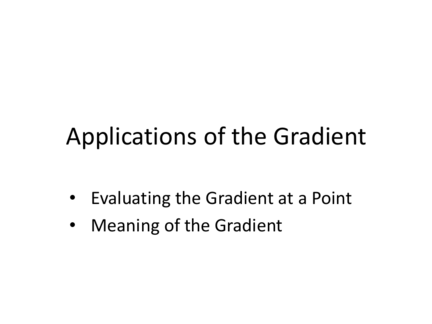Contributed by:This pdf includes the following topics:-
Practice Problems 1 and 2
Examples
• Evaluating the Gradient at a Point
In 1-variable calculus, the derivative gives you an
equation for the slope at any x-value along f(x).
You can then plug in an x-value to find the actual
slope at that point. 2 f(x) = x
f’(x) = 2x
Actual tangent line slope is… 10 when x = 5
-4 when x = -2 5 when x = 2.5
0 when x = 0
Similarly, the gradient gives you an equation for
the slope of the tangent plane at any point (x, y)
or (x, y, z) or whatever. You can then plug in the
actual values at any point to find the slope of
the tangent plane at that point.
The slope of the tangent plane will be written as
a vector, composed of the slopes along each
As an example, given the function f(x, y) = 3x2y –
2x and the point (4, -3), the gradient can be
calculated as:
[6xy – 2 3x2]
Plugging in the values of x and y at (4, -3) gives
[-74 48]
which is the value of the gradient at that point.
5. Practice Problems 1 and 2
1. f(x, y) = x2 + y2 at
a) (0, 0) b) (1, 3) c) (-1, -5)
2. f(x, y, z) = x3z – 2y2x + 5z at
a) (1, 1, -4) b) (0, 1, 0) c) (-3, -2, 1)
In the previous example, the function f(x, y) =
3x2y – 2x had a gradient of [6xy – 2 3x2], which
at the point (4, -3) came out to [-74 48].
500
The tangent plane at that
400
300
200
100 (4, -3) point will have a slope of
0
-100
-200
-300
-400
-74 in the x direction and
-500
-600
-700
-800
+48 in the y direction.
3 -1
y axis x axis
-5 7
Even more important is the vector itself, [-74 48].
Here is the graph again, with the vector drawn in
as a vector rather than two sloped lines:
Recall that vectors give us
500
400
300
direction as well as magnitude.
200
100
0
-100
-200
-300
-400
-500
-600
-700
vector will always point in the
3
-800
-1 direction of steepest increase for
y axis x axis
-5 7 the function.
And, its magnitude will give us the slope of the plane in
that direction.
That’s a lot of different slopes!
16 Each component of the gradient
14
vector gives the slope in one
12
10
dimension only.
8
6
4
vector gives the steepest possible
2 5
4
slope of the plane.
3
0 2
0 1
1 2 0
3 4 5 6
Recall that the magnitude can be found using the Pythagorean
Theorem, c2 = a2 + b2, where c is the magnitude and a and b are
the components of the vector.
9. Practice Problem 3
Find the gradient of f(x, y) = 2xy – 2y, and the
a) (0, 0) 40
30
b) (5, -3) 20
10
0
-10
-20
c) (20, 10) -30
-40
d) (-5, 4)
e) Find where the gradient = 0.
10. Practice Problem 4
f(x, y, z, w) = 3xy – 2xw + 5xz – 2yw
and the magnitude of the gradient at (0, 1, -1, 2).
11. Practice Problem 5
Suppose we are maximizing the function
f(x, y) = 4x + 2y – x2 – 3y2
Find the gradient and its magnitude from
a) (1, 5)
b) (3, -2)
c) (2, 0)
d) (-4, -6)
e) Find where the gradient is 0.
12. Practice Problem 6
Suppose you were trying to minimize f(x, y) = x2
+ 2y + 2y2. Along what vector should you travel
from (5, 12)?